# ISEE Upper Level Math : How to find the area of an acute / obtuse triangle

## Example Questions

### Example Question #1 : How To Find The Area Of An Acute / Obtuse Triangle

Two sides of a scalene triangle measure 4 centimeters and 7 centimeters, and their corresponding angle measures 30 degrees. Find the area of the triangle.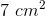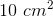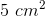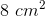Explanation: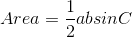,

whereandare the lengths of two sides andis the angle measure.

Plug in our given values: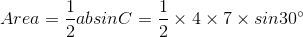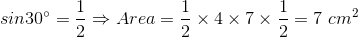### Example Question #2 : How To Find The Area Of An Acute / Obtuse Triangle

A scalene triangle has a base lengthand a corresponding altitude of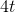. Give the area of the triangle in terms of.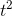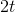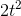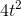Explanation: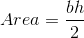,

whereis the base andis the altitude.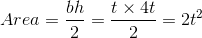### Example Question #3 : How To Find The Area Of An Acute / Obtuse Triangle

What is the area of a triangle on the coordinate plane with its vertices on the points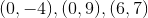?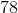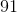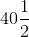Explanation:

The base can be seen as the (vertical) line segment connecting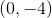and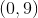, which has length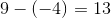. The height is the pependicular distance from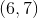to the segment; since the segment is part of the-axis, this altitude is horizontal and has length equal to-coordinate.

The area of this triangle is therefore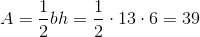.

### Example Question #4 : How To Find The Area Of An Acute / Obtuse Triangle

What is the area of a triangle on the coordinate plane with its vertices on the points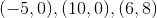?Explanation:

The base can be seen as the (horizontal) line segment connecting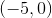and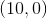, the length of which is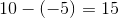. The height is the pependicular distance from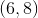to the segment; since the segment is part of the-axis, this altitude is vertical and has a length equal to-coordinateThe area of this triangle is therefore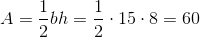.

### Example Question #5 : How To Find The Area Of An Acute / Obtuse Triangle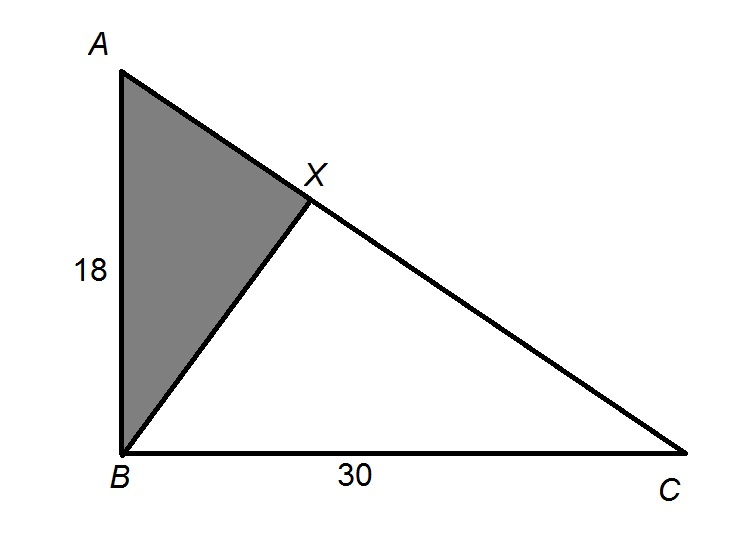Figure NOT drawn to scale.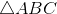is a right triangle with altitude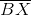. What percent ofhas been shaded gray?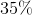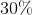Explanation:

The altitude of a right triangle from the vertex of its right angle - which, here, is- divides the triangle into two triangles similar to each other as well as the large triangle.

The similarity ratio of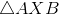tois the ratio of the lengths of their hypotenuses. The hypotenuse of the latter is 18; that of the former, from the Pythagorean Theorem, is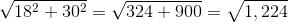The similarity ratio is therefore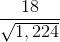. The ratio of their areas is the square of this, or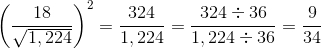The area ofis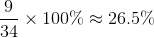of that of, so the choice closest to the correct percent is 25%.